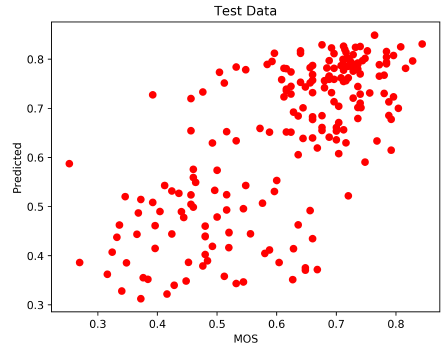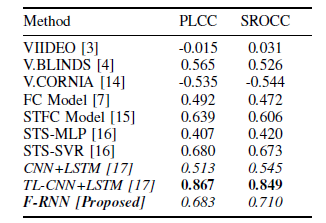# Review — F-RNN: No-Reference Video Quality Assessment using Recurrent Neural Networks

## F-RNN, Combining Frame-Level Features Using RNN for NR-VQA

In this story, No-Reference Video Quality Assessment using Recurrent Neural Networks, (F-RNN), by Sharif University of Technology, is reviewed.

• Due to the duration and the excessive number of the videos, case by case assessment of the videos by operators is no longer feasible.
• No-reference (Blind) video quality assessment (NR-VQA) technique is used for automatically assessing the video quality. However, many prior arts NR-VQA techniques are insensitive to the frame order.
• In this paper, F-RNN is proposed where its RNN is responsible to combine frame-level features by preserving their order so as to form a single video quality metric.

This is a paper in 2019 ICSPIS. (Sik-Ho Tsang @ Medium)

# Outline

1. Frame-Level Features
2. F-RNN: Network Architecture
3. Experimental Results

# 1. Frame-Level Features

## 1.1. Luminance MSCN Statistics

• Let F(i, j) represent the luminance values of a given frame in the video at (i, j). The Mean Subtracted Contrast Normalized (MSCN) luminance values are defined as:
• where μ is the local mean and σ is the local standard deviation, The constant C is conventionally set as 1 to avoid instability:
• The weights w = {wk,l | k = -K, …, K; l = -L, …, L} are obtained from a 2D circularly-symmetric Gaussian function:
• where K=L=3.
• Previous studies in ,  show that the histogram of ^F(i, j) can be fairly described by a Generalized Gaussian Distribution (GGD):
• where Τ() is the gamma function.
• Moreover, the pair-wise product of the adjacent pixels in ^F(i, j) fairly follow an Asymmetric Generalized Gaussian Distribution (AGGD):
• The mean of this distribution is given by:
• The parameters (α, β), and (γ, βl, βr, η) for four orientations of pairing form a total of 18 statistical parameters.
• By considering the image in two scales, the original one and a factor two down-sampled, a total of 36 statistical parameters is formed.
• (I think this part should refer to  and  for better understanding.)

## 1.2. Luma Information

• The mean and standard deviation of the luminance channel are estimated. 2 parameters are formed.

## 1.3. Chroma Information

• The mean and standard deviation of each chrominance channel are estimated. 4 parameters are formed.

## 1.4. Colorfulness

• The introduced Chroma information somehow presents the distribution of color intensities within the video.
• Another metric for measuring the colorfulness of images is presented in . Using RGB space, we have:
• The colorfulness metric M^(3) in  is defined as:
• where
• The value of M^(3) for every frame of the video is estimated.
• 1 parameter is formed.

## 1.5. Spatial Gradient

• The horizontal and vertical gradients (derivatives) of this image reveal the edges.
• Two 5×5 filter kernels (horizontal and vertical directions) on the luminance channel of the frame. Next, we compute the mean and standard deviation for each frame. 4 parameters are formed.

## 1.6. Spatial Laplacian

• The Laplacian of a frame is a particular 2nd order derivative of the data and is known to be rotation and scale-invariant.
• The Laplacian operator via a 5×5 filter kernel is applied to the luminance channel of the frame. The mean and standard deviation of the result over each frame are estimated. 2 parameters are formed.

## 1.7. Temporal Information

• Difference frames are generated by subtracting the luminance channel of each frame from the luminance channel of the preceding frame.
• Difference frames are converted into features by evaluating the mean and standard deviation of each difference frame. 2 parameters are formed.

In Brief, 51 handcrafted features are obtained based on some prior arts, as well as some standard statistics such as mean and standard deviations.

In total, there are 36+2+4+1+4+2+2=51 features are obtained.

# 2. F-RNN: Network Architecture

• 51 features are firstly normalized, and fed to a recurrent neural network (RNN) in a sequential manner.
• Three Bidirectional-LSTM units followed by two fully connected layers are used.
• In each of the fully connected layers, batch normalization is applied before the tanh activation function.
• Eventually, the output neuron with logistic sigmoid function is employed to predict the overall video quality in the range (0,1).
• MSE is used to train the RNN.
• Batch size of 64 is used.
• Dropout with p = 1/2 is used in the Bi-LSTM and fully connected layers.
• The training procedure with 100 epochs took about one hour.

# 3. Experimental Results

## 3.1. Dataset

• KonVid-1k database is used, where it got 1200 human-rated video files.
• Each video in this database is accompanied with around 50 human subjective scores.
• The length of each video in KonVid-1k database are not the same. However, this database contains a subset of 810 videos with 240 frames. For the purpose of training and testing the network, only the subset of size 810 (i.e., ignoring the remaining 390 files in the database) is considered.
• 75% of this subset is randomly selected; Among them, 80% are used for training, 20% are used for validation.
• Finally, we use the rest (25%) of the subset for testing the trained RNN.

## 3.2. ResultsThe scatter plot of quality scores of test videos
• The horizontal axis indicates the ground-truth MOS and the vertical axis indicates the predicted MOS.
• This shows the linearity of the prediction using RNN.The Pearson linear correlation coefficient (PLCC) and Spearman rank order correlation coefficient (SROCC)
• The proposed F-RNN achieves comparable or better results, while employing a simple architecture.
• The features used in this paper are simple and fast to extract.
• Authors believe that more detailed features can lead to performance gains using the same RNN structure.
• Another possible direction for improvement is to combine the convolutional neural networks (CNNs) and RNNs; in particular, the CNN provides richer feature sets while the RNN provides a smart aggregation technique.

## Reference

[2019 ICSPIS] [F-RNN]
No-Reference Video Quality Assessment using Recurrent Neural Networks

## Video Quality Assessment (VQA)

FR: [DeepVQUE]
NR: [SACONVA] [3D-CNN+LSTM] [F-RNN]

--

--